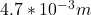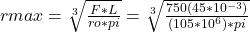Question

A circular specimen of MgO is loaded using a three-point bending mode. Compute the minimum possible radius of the specimen without fracture, given that the applied load is 750 N (169 lbf), the flexural strength is 105 MPa (15,000 psi), and the separation between load points is 75.0 mm (2.95 in.).

1.thanhcong

The answer isrmax =